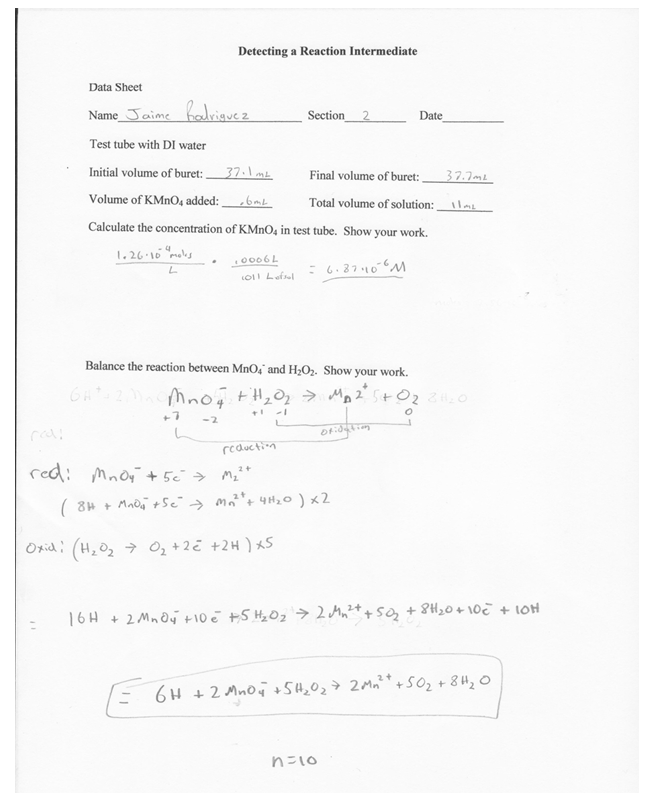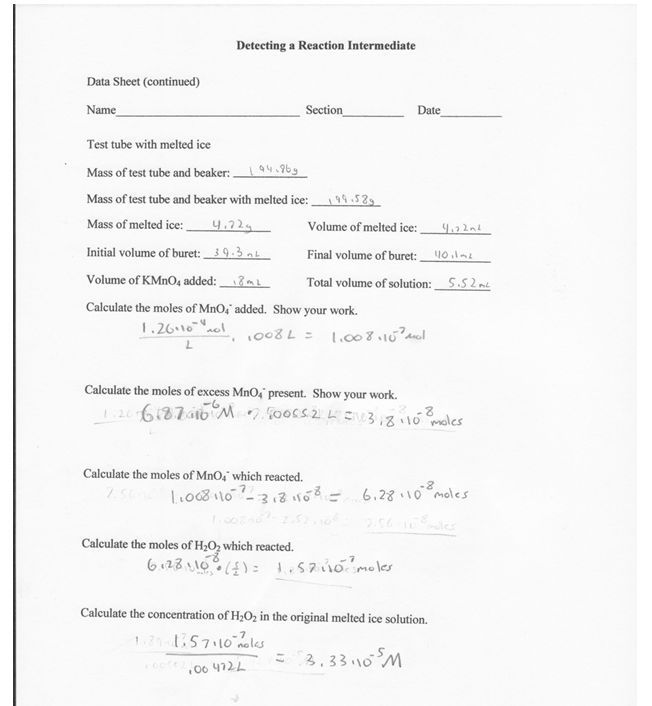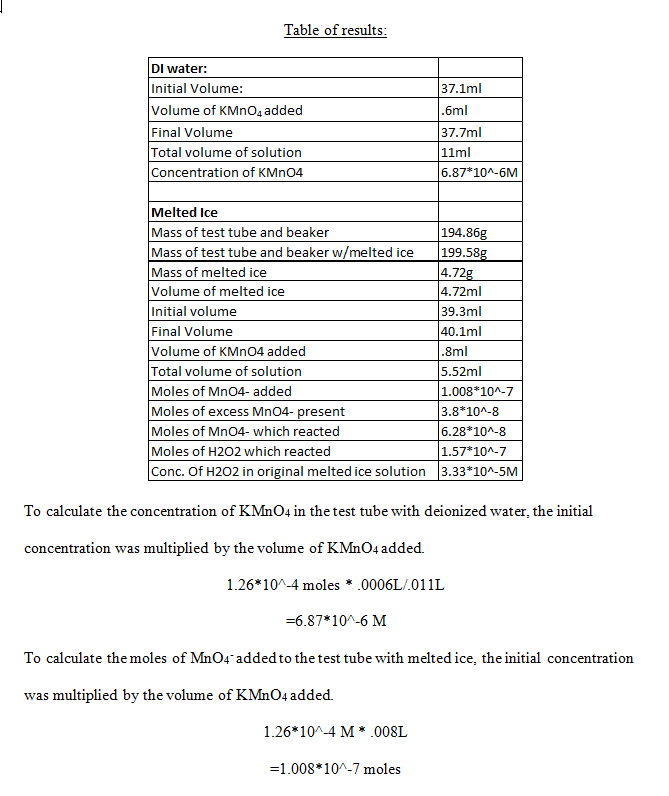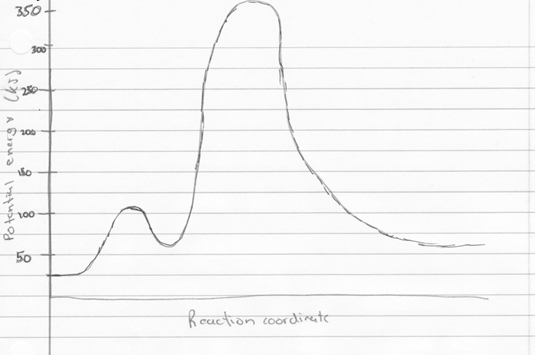# Determining a Reaction Intermediate

By: Jaime Rodriguez

Background:

In this experiment, the concentrations of KMnO4 in each test tube were considered similar if their respective colors were similar. This step is what made it possible to calculate the amount of hydrogen peroxide in the original solution of melted ice. Unfortunately, the human eye is not keen to accurately detect the change in color intensity; therefore the colors in both test tubes may not have been very similar at all. Besides this method, there is another, more accurate, method that could determine when all of the hydrogen peroxide was consumed in the reaction. With the use of a ph monitor, the total consumption of the hydrogen peroxide could be determined. ( ph of H2O2 Solutions). In the reaction, the addition of the hydrogen peroxide turns the solution from basic to acidic. After all of the hydrogen has been consumed, the solution will return to a base. If the shifts in ph are monitored, it can accurately be determines when all of the hydrogen peroxide has been consumed.

Procedure:

No changes were made to the procedure. It was followed as it was written.

Data and Results:Table of results:The number of moles of excess MnO4 present was calculated by taking the concentration of KMnO4 from the test tube with deionized water, and multiplying it by the total volume of solution in the test tube with melted ice.

6.87*10^-7M * .00552L

=3.8*10^-8 moles

The number of moles of MnO4 which reacted was found simply by subtracting the number of moles in excess by the number of moles added.

1.008*10^-7 moles  – 3.8*10^-8 moles

=6.28*10^-8 moles

The moles of H2O2 which reacted was found by using the stoichiometric ratio of number of moles of H2O2  to number of moles of MnO4 .

6.28*10^-8 moles of MnO4* (5 moles of H2O2/2 moles of MnO4)

=1.57*10^-7 moles

Finally, the concentration of H2O2  in the original melted ice solution was calculated by dividing the number of moles of H2O2  which reacted by the volume of melted ice.

1.57*10^-7 moles/.00472L

=3.33*10^-5 M

The reaction between H2O2  and MnO4 might proceed more slowly at the end because by this time, almost all of the H2O2  is being consumed in the reaction. The concentration of hydrogen peroxide that was measured was 3.33*10^-5 M

To calculate the free energy of the reactions for hydrogen gas reacting with gaseous water to form gaseous hydrogen peroxide, and gaseous hydrogen peroxide reacting with hydrogen gas to form water, were found by using the Gibbs free energy equation.

The equation states that,  delta G=delta H-delta S(T). To find delta S and H, the values of H and S for each of the reactants and products were found. Then, the sum of the products was subtracted by the sum of the reactants. Once this was done, the values were put in to the Gibbs free energy equation, and the temperature used was 198.15 K, room temperature. The free energy values were -105.5kJ, and -351.5kJ, respectively. The free energy values for these two equations should be equivalent to the free energy associated with the combustion of hydrogen, since both are intermediate reactions, with hydrogen peroxide being the reaction intermediate. Below is the corresponding potential energy diagram.Reflection

The experiment was easy, relatively quick, and yielded good quality data. Some of the calculations got confusing, but they were eventually figured out. There were really no issues with the experiment, and it was overall relatively enjoyable.

References:

pH of H2O2 solutions? | H2O2.com – US Peroxide – Technologies for Clean Environment.” US Peroxide – Technologies for a Clean Environment. N.p., n.d. Web. 22 Apr. 2013. <http://www.h2o2.com/faqs/FaqDetail.aspx?fId=26>.

• Share